International
Tables for
Crystallography
Volume A
Space-group symmetry
Edited by M. I. Aroyo

International Tables for Crystallography (2016). Vol. A, ch. 3.6, pp. 863-864

## Section 3.6.5. Maximal subgroups of index ≤ 4

D. B. Litvina*

aDepartment of Physics, The Eberly College of Science, Penn State – Berks Campus, The Pennsylvania State University, PO Box 7009, Reading, PA 19610–6009, USA
Correspondence e-mail: u3c@psu.edu

### 3.6.5. Maximal subgroups of index ≤ 4

| top | pdf |

We consider the maximal subgroups of index ≤ 4 of the one-, two- and three-dimensional magnetic space groups and the two- and three-dimensional magnetic subperiodic groups. A complete listing of the maximal subgroups of the two- and three-dimensional non-primed space groups can be found in International Tables for Crystallography, Volume A1, Symmetry Relations Between Space Groups (2010; IT A1). The maximal subgroups of index ≤ 4 of the three-dimensional non-primed space groups and non-primed layer and rod groups can also be found on the Bilbao Crystallographic Server (http://www.cryst.ehu.es ; Aroyo et al., 2006).

For magnetic groups, an abstract of a method for determining the maximal subgroups of magnetic groups was published by Sayari & Billiet (1977). The maximal subgroups of magnetic groups found in Litvin (2013) were derived from Litvin (2008a) using a method given by Litvin (1996).

Each maximal subgroup table is headed by the magnetic group type whose maximal subgroup types are to be listed.

#### Examples

For the three-dimensional magnetic space group type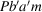, one finds (Litvin, 2013), in bold blue type, information which defines the representative group of this type in a coordinate system O; a, b, c: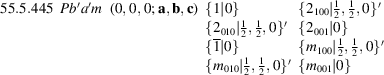The first column gives the global serial number of the group, followed in the second column by its magnetic group type symbol. In the third column, the symbol (O; a′, b′, c′) gives the origin O and basis vectors a′, b′, c′ of the conventional unit cell of the non-primed subgroup of the representative group of the type Pbam. These basis vectors a′, b′, c′ imply both the magnetic and non-primed translational subgroups of the representative group. In this case, the P translational subgroup of the representative group is non-primed and generated by the basis vectors a, b, c. The standard set of coset representatives of this representative group is given on the right.

For the three-dimensional magnetic space-group type P2bma2 one finds (Litvin, 2013):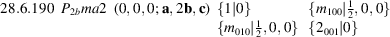Note here that a′ = a, b′ = 2b, c′ = c, being the basis vectors of the conventional unit cell of the non-primed subgroup of the representative group of P2bma2, implies that the translational subgroup of the representative group is P2b, i.e. generated by the non-primed translations {1∣1, 0, 0}, {1∣0, 2, 0}, {1∣0, 0, 1} and the magnetic translation {1∣0, 1, 0}′.

Following the subtable heading of each magnetic space-group type is a listing of the maximal subgroups of index ≤ 4 of the representative magnetic space group of this type.

#### Examples

From the list of maximal subgroups of the representative magnetic group of the type Pbam is the subgroup listed as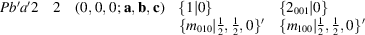The first column gives the magnetic group type symbol of the subgroup. The second column gives the subgroup index of this subgroup as a subgroup of the representative group of type Pbam. In the third column, the symbol (O + p; a′, b′, c′) gives the origin `O + p' and basis vectors a′, b′, c′ of the conventional unit cell of the non-primed subgroup, where p, a translation of the origin of the coordinate system of the representative group Pbam, and the conventional unit cell are such that the coset representatives listed are transformed into the standard cosets of the representative group of the subgroup type Pba′2. In this case, since the listed coset representatives are the standard cosets of the representative group of type Pba′2, no translation of origin is required, and consequently O + p = 0, 0, 0. The conventional unit cell of the non-primed subgroup of the subgroup Pba′2 is the same as that of representative group of the type Pba′2 and consequently one finds a′ = a, b′ = b, c′ = c.

That the listed coset representatives of the subgroup are the same as those of the representative group of that subgroup type is not always the case:

#### Example

A subgroup of type P2/m of the representative group Pbam is listed as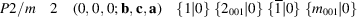The standard set of coset representatives of the representative group P2/m are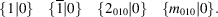A change in setting to have the coset representatives of the subgroup be identical with the coset representatives of the representative group P2/m is represented in the symbol (0, 0, 0; b, c, a), i.e. changing the setting from a, b, c to b, c, a.

Other cases may require a simultaneous change to both the origin and the setting of the conventional unit cell of the non-primed subgroup:

#### Example

A third subgroup of the representative group Pbam is the equi-class subgroup of the same type Pbam: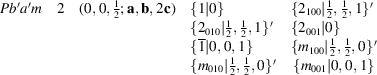The listed coset representatives of this subgroup are not the same as the coset representatives of the representative group Pbam, i.e. where the z component of the non-primitive translation associated with all coset representatives is zero. To have these listed coset representatives become identical with the coset representatives of the standard representative group of Pbam, one must change the origin of the coordinate system. This information is provided in the symbol (0, 0, ½; a, b, 2c) where O + p = 0, 0, ½ denotes the translation under which all the non-zero z components of the coset representatives are transformed to zero. Note also that the P in the subgroup symbol denotes a non-primed translational subgroup which is determined by a′ = a, b′ = b, c′ = 2c, i.e. P denotes the translational group generated by the translations {1∣1, 0, 0}, {1∣0, 1, 0} and {1∣0, 0, 2}.

In the tabulations of the maximal subgroups of groups of the type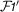not all maximal subgroups are explicitly listed. The maximal subgroup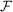ofis not listed. Ifis a maximal subgroup of, then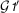is a maximal subgroup ofand is also not explicitly listed. All maximal subgroupsofare listed under, and consequently, all maximal subgroupsofare then found from the list of all maximal subgroupsofby multiplying each by. For each listed maximal subgroup, its non-primed subgroup type is explicitly given. For example, a listed subgroup of Pma21′ is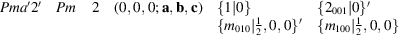where the non-primed subgroup type Pm of Pma′2′ is given in the second column.

### References

International Tables for Crystallography (2010). Vol. A1, Symmetry Relations Between Space Groups, 2nd ed., edited by H. Wondratschek & U. Müller. Chichester: Wiley. [First edition 2004.]
Aroyo, M. I., Perez-Mato, J. M., Capillas, C., Kroumova, E., Ivantchev, S., Madariaga, G., Kirov, A. & Wondratschek, H. (2006). Bilbao Crystallographic Server I: Databases and crystallographic computing programs. Z. Kristallogr. 221, 15–27.
Litvin, D. B. (1996). Maximal subgroups of magnetic space groups and subperiodic groups. Acta Cryst. A52, 681–685.
Litvin, D. B. (2008a). Magnetic group maximal subgroups of index 4. Acta Cryst. A64, 345–347.
Litvin, D. B. (2013). Magnetic Group Tables, 1-, 2- and 3-Dimensional Magnetic Subperiodic Groups and Space Groups. Chester: International Union of Crystallography. Freely available from http://www.iucr.org/publ/978–0–9553602–2–0 .
Sayari, A. & Billiet, Y. (1977). Subgroups and changes of standard setting of triclinic and monoclinic space groups. Acta Cryst. A33, 985–986.